# Physical Chemistry : Thermochemistry

## Example Questions

### Example Question #21 : Thermochemistry And Thermodynamics

What amount of heat must be added to a 20-gram sample of ice in order to raise the temperature from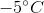to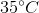?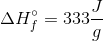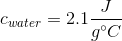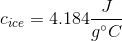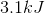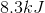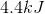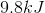Explanation:

This question requires three steps:

1. We need to raise the temperature of the ice fromtodegrees Celsius.

2. We need to melt the ice.

3. We need to raise the temperature of the water fromtodegrees Celsius.

Steps one and three can use the equation: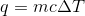Step 2 requires the enthalpy of fusion to be multiplied by the mass of the water sample.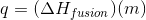Finding these three added heats will give us the total amount of heat needed to raise the temperature fromto.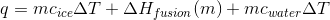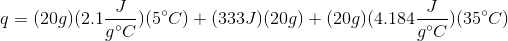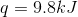### Example Question #1 : Thermochemistry

What is the process of converting a solid directly to a gas?

Sublimation

Freezing

Deposition

Condensation

Sublimation

Explanation:

Certain substances, such as carbon dioxide, are able to skip the liquid phase and go from a solid to a gas under standard conditions. This process is called sublimation.

### Example Question #1 : Heat Capacity And Specific Heat

What is the specific heat capacity for a 50-gram sample of metal that increases in temperature by 10 degrees celsius when 2000 joules of energy is added?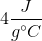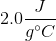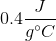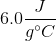Explanation:

Use the equation: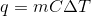We can calculate the specific heat capacity for the unknown metal. Since we know the added heat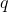, the mass of the sample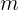, and the change in temperature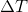, we can solve for.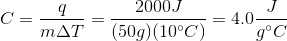### Example Question #1 : Heat Capacity And Specific Heat

A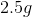sample of an unknown metal atis immersed in water and is allowed to reach equilibrium. If final temperature of the system is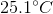and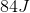of energy are released, what is the identity of the metal?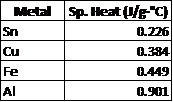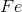It is impossible to determine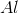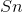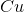Explanation:

Recall that the formula for specific heat is. Rearranging the equation for specific heat (c) yields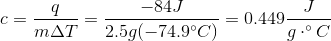Remark: keep in mind that the release of energy and the cooling of the metal will give negative values for bothand### Example Question #21 : Physical Chemistry

The heat capacity of a bomb calorimeter assembly is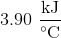. What is the heat of combustion of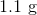of sucrose in a bomb calorimeter that results in the temperature rising from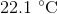to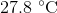?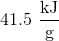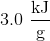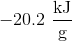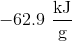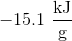Explanation:

A bomb calorimeter is a device used to measure the quantity of heat change for a process. The heat of a reaction which is denoted as, is the negative of the thermal energy gained by the calorimeter: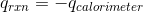The heat capacity of a calorimeter is: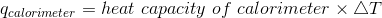Plugging the values given into the equation gives: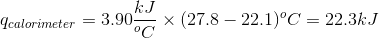Using the relation provided earlier: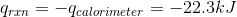Because we are dealing with 1.1 grams of sucrose, the heat of combustion of sucrose is: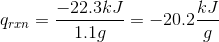### Example Question #21 : Physical Chemistry

The heat capacity of a bomb calorimeter assembly is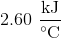. What is the heat of combustion of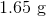of caffeine in a bomb calorimeter that results in the temperature rising from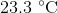to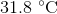?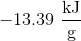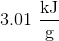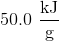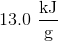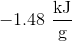Explanation:

A bomb calorimeter is a device used to measure the quantity of heat change for a process. The heat of a reaction which is denoted as q, is the negative of the thermal energy gained by the calorimeter:The heat capacity of a calorimeter is:Plugging the values given into the equation gives: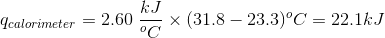Using the relation provided earlier: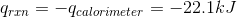Because we are dealing with 1.65 grams of sucrose, the heat of combustion of sucrose is: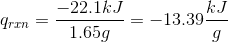### Example Question #1 : Thermochemistry

Which of the following are endothermic?

Condensation

Melting

Freezing

More than one of these are endothermic processes

Melting

Explanation:

Endothermic processes involve a positive change in enthalpy. This means that the enthalpy of products is higher than the enthalpy of reactants and net heat energy is consumed. Phase changes that involve increasing the distance between particles (meaning conversion of solid to liquid (melting), liquid to gas (evaporation) and solid to gas (sublimation)) require an input of energy and are considered endothermic processes. On the other hand, phase changes that decrease the distance between particles (such as gas to liquid (condensation), liquid to solid (freezing), and gas to solid (deposition)) release energy and are considered exothermic processes.

### Example Question #1 : Thermochemistry

Which of the following is true regarding an exothermic reaction?

I. Entropy always increases

II. It is always spontaneous

III. It can facilitate other energy-consuming reactions

I and II

I and III

II only

III only

III only

Explanation:

Exothermic reactions involve release of heat energy. This means that the energy of the products is lower than the energy of the reactants. Entropy is the measure of disorder in a system. It does not depend on the enthalpy and can increase or decrease during an exothermic process. Spontaneity is determined by looking at the change in Gibbs free energy,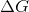. Negativecorresponds to a spontaneous process and positivecorresponds to a nonspontaneous process. While the equation for Gibbs free energy,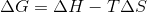, involves change in enthalpy, the spontaneity also depends on temperature and change in entropy. Exothermic reactions release energy in the form of heat. This heat energy can be used to power other processes that require energy; therefore, exothermic reactions can facilitate active processes that consume energy.

### Example Question #1 : Endothermic And Exothermic Reactions

In an __________ reaction, the products are more stable than the reactants; in an __________ reaction the reactants are more stable than the products.

exergonic . . . endergonic

endergonic . . . endergonic

exergonic . . . exergonic

endergonic . . . exergonic

exergonic . . . endergonic

Explanation:

Exergonic reactions release energy; therefore, the energy of products is lower than that of the reactants. Endergonic reactions consume energy; therefore, the energy of products is greater than that of the reactants. In other words, exergonic reactions are spontaneous, while endergonic reactions are nonspontaneous, and require the net input of energy to drive the reaction.

### Example Question #1 : Thermochemistry

Consider the following spontaneous reaction: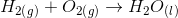What can you conclude about the enthalpy change in this reaction?

It is zero

Cannot be determined from the given information

It is positive

It is negative

It is negative

Explanation:

We need to use the following equation to answer this question:Above,is change in Gibbs free energy,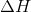is change in enthalpy,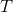is temperature, and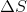is change in entropy. The question states that the reaction is spontaneous; therefore,is negative. We can also determine thefor this reaction by looking at the phases of the products and reactants. Recall that entropy is a measure of disorder. When it comes to phases, gases have the highest entropy and solids have the lowest entropy. This is because in gases the molecules are spread out and have more room for disorder while solids are compressed and well packaged, decreasing the disorder of the atoms/molecules. Liquids are intermediate in entropy. In this reaction, we are creating a liquid from two molecules of gas; therefore, we are decreasing the entropy of the system (going to a more ordered, liquid state). The change in entropy for this reaction is negative.

Rearranging and solving the equation above forgives us: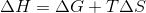Since bothandare negative,will always be negative (regardless of temperature). This is an exothermic reaction.

### All Physical Chemistry Resources